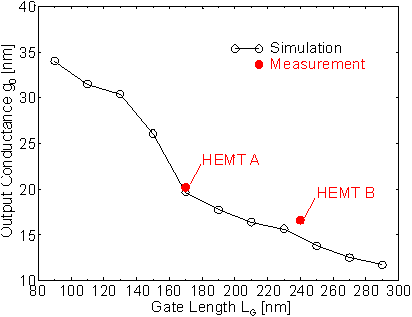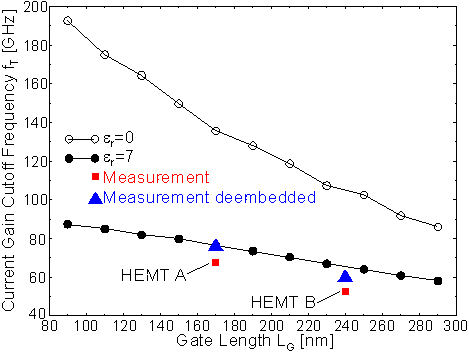Next: 6.1.2.2 Contributions to the Gate Capacitance Up: 6.1.2 RF Characteristics Previous: 6.1.2 RF Characteristics

6.1.2.1 Reduction of the Gate Length

When LG is reduced, fT will increase, but a simultaneous and undesirable increase of the output conductance g0 must be expected due to short channel effects such as increased real space transfer. In  Figure 6.14 the simulated parameter g0 (at the bias point VDS = 2 V, VGS = 0.2 V) is shown as a function of LG and compared to the experimental DC values of HEMTs A and B which differ only with respect to LG but not in any other geometrical dimensions. The simulation is able to reproduce g0 realistically.Figure 6.14 Measured (filled symbols) and simulated (open symbols) DC output conductance g0 versus the gate length at the bias point VGS = 0.2 V and VDS = 2.0 V

As described in Section 5.3.1, the increase of gm ext is only small when LG is reduced. The capacitance CGD is nearly independent of LG. The gate-source capacitance CGS is only partly dependent on LG: fringe and other parasitic contributions are independent of LG, only the part due to the gate contact area is length dependent. Therefore, the improvement of fT which can be achieved by a reduction of LG depends on the relative contribution of the parasitic (i. e. constant) part of the capacitance to the gate capacitance CG.

In  Figure 6.15 the simulated fT is shown along with the measured and deembedded fT according to the procedure described in Section 3.2. Although only the simulation of HEMT A was fitted to the deembedded fT also the fT of HEMT B is simulated very well. The measured values in  Figure 6.15 (which are taken from HEMTs A and B) are even below the ones calculated with complete T-gate structure and passivation nitride due to the presence of parasitic pad capacitances as discussed before.Figure 6.15 Measured (filled squares) and simulated fT versus LG for a passivated HEMT with er = 7 (filled circles) and er = 0 (open circles) at VGS = 0.2 V and VDS = 2.0 V

The dependence of fT on LG is shown in  Figure 6.15 for two examples: the theoretical limiting case of er = 0 where the contribution of parasitics is reduced to the inevitable fringe capacitances at the gate edge, and the case of the presence of a medium with er = 7 (a silicon nitride passivation layer, for instance) which fills the space between the T­gate overhang and the semiconductor surface in the manner sketched in  Figure 6.6. Figure 6.15 demonstrates clearly that the improvement of fT achieved by a reduction of LG depends strongly on the relative contribution of parasitic capacitive couplings to the total gate capacitance. The reduction of fT in the presence of passivation layers has been experimentally observed by Wu et al. .

To obtain an fT of 100 GHz, a fully passivated device of the general structure of HEMT A must be either supplied with a gate length below 100 nm, or the gate length is left as large as in HEMT B (240 nm) but no passivation is allowed at all. The case of an unpassivated device in air with er = 1 is close to the idealized case er = 0 plotted in  Figure 6.15. The two curves in the Figure are calculated under the assumption of a constant interface charge density which is certainly an idealization when the unpassivated case is considered.Next: 6.1.2.2 Contributions to the Gate Capacitance Up: 6.1.2 RF Characteristics Previous: 6.1.2 RF Characteristics

Helmut Brech
1998-03-11